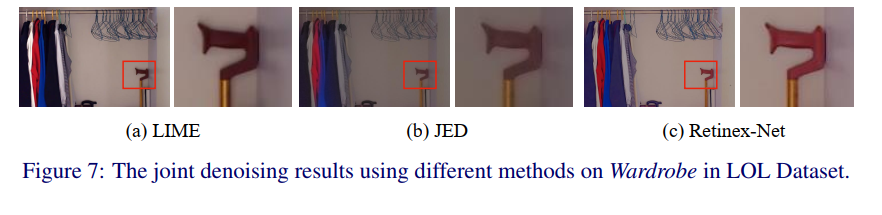> 文档中心 > RetinexNet: Deep Retinex Decomposition for Low-Light Enhancement

# RetinexNet: Deep Retinex Decomposition for Low-Light Enhancement

### 文章目录

• 摘要
• 1、简介
• 2、光增强RetinexNet
• 2.1 数据驱动的图像分解
• 2.2 结构感知平滑性损失
• 2.3 多尺度照明调节
• 2.4 反射率去噪
• 3、数据集
• 3.1 真实场景采集数据集
• 3.2 从原始图像合成图像对
• 4.2 分解结果
• 4.3 评价
• 4.4 微光增强和去噪联合
• 5、结论

# 摘要

Retinex模型是微光图像增强的有效工具。假设观测图像可以分解为反射率和照度。大多数现有的基于retina的方法都为这种高度病态分解精心设计了手工制作的约束和参数，在应用于各种场景时可能会受到模型容量的限制。在本文中，我们收集了一个包含低光/正常光图像对的low -light数据集(LOL)，并提出了一个基于该数据集的深度视网膜网，包括一个用于分解的分解网(Decom-Net)和一个用于亮度调整的增强网(enhanced - net)。在Decom-Net的训练过程中，没有分解反射率和光照的ground truth。该网络的学习只有关键约束，包括由配对的低光/正常光图像共享的一致反射率，以及照明的平稳性。在此基础上，通过增强网络(Enhance-Net)对光照进行亮度增强，联合去噪则对反射率进行去噪。视网膜网是端到端可训练的，因此学习到的分解本质上有利于亮度调整。大量的实验证明，我们的方法不仅在弱光增强中获得了视觉上令人愉悦的质量，而且还提供了良好的图像分解表示。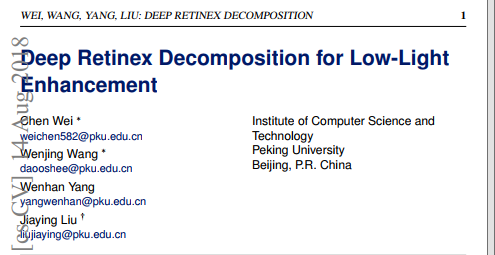# 1、简介

• 我们建立了一个大规模的数据集与配对的低/正常光图像捕获的真实场景。据我们所知，这是在微光增强领域的第一次尝试。

• 构建了基于Retinex模型的深度学习图像分解。分解网络与连续弱光增强网络进行端到端训练，因此框架具有良好的光条件调整能力。

• 我们提出了一种结构感知的全变分约束用于深度图像分解。通过在梯度较强的地方减少总变化的影响，该约束成功地平滑了照明图并保留了主要结构。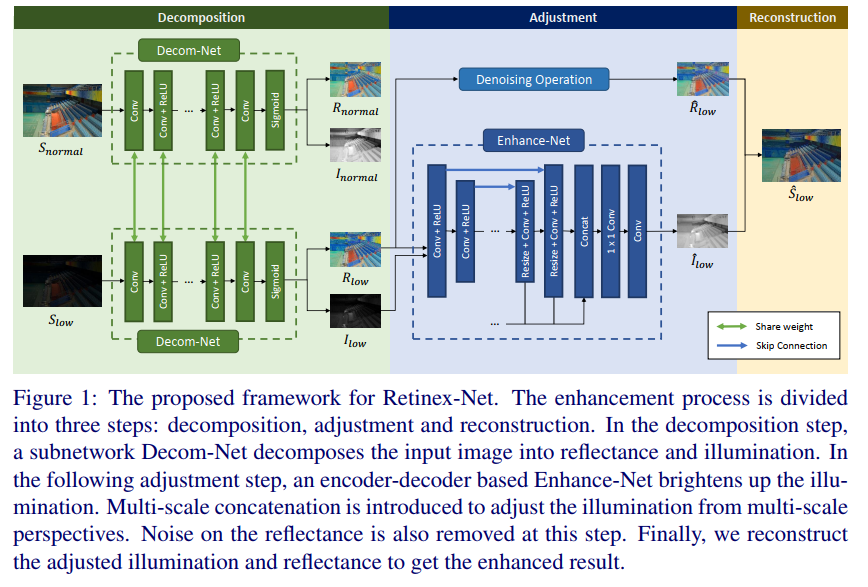# 2、光增强RetinexNet

S = R ∘ I (1) S=R \circ I \tag{1}

## 2.1 数据驱动的图像分解

L = L recon  + λ i r L i r + λ i s L i s , (2) \mathcal{L}=\mathcal{L}_{\text {recon }}+\lambda_{i r} \mathcal{L}_{i r}+\lambda_{i s} \mathcal{L}_{i s}, \tag{2}

L recon  = ∑ i =  low,normal  ∑ j =  low,normal  λ i j ∥ R i ∘ I j − S j ∥ 1 .  (3) \mathcal{L}_{\text {recon }}=\sum_{i=\text { low,normal }} \sum_{j=\text { low,normal }} \lambda_{i j}\left\|R_{i} \circ I_{j}-S_{j}\right\|_{1} \text {. } \tag{3}

Lir  = ∥ R low − R normal ∥ 1 . \mathcal{L}_{\text {ir }}=\left\|R_{\text {low }}-R_{\text {normal }}\right\|_{1} .

## 2.2 结构感知平滑性损失

L i s = ∑ i =  low,normal  ∥ ∇ I i ∘ exp ⁡ ( − λ g ∇ R i ) ∥ , (5) \mathcal{L}_{i s}=\sum_{i=\text { low,normal }}\left\|\nabla I_{i} \circ \exp \left(-\lambda_{g} \nabla R_{i}\right)\right\|, \tag{5}

## 2.3 多尺度照明调节

L recon  = ∥ R low  ∘ I ^ − S normal  ∥ 1 . (6) \mathcal{L}_{\text {recon }}=\left\|R_{\text {low }} \circ \hat{I}-S_{\text {normal }}\right\|_{1} .\tag{6}

Lis \mathcal{L}_{i s} 与Eq.(5)相同，只是 i ^ \hat{i} R low R_{\text {low}} 的梯度映射加权。

# 3、数据集

## 3.2 从原始图像合成图像对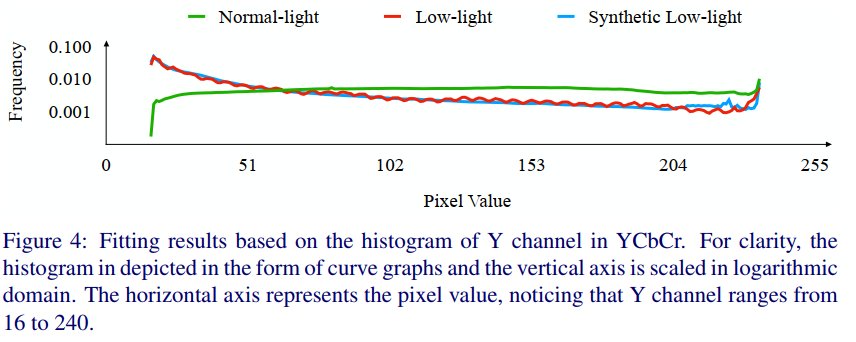## 4.2 分解结果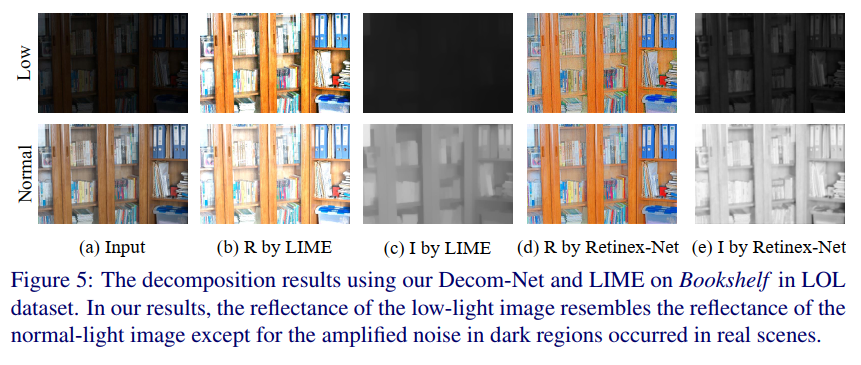## 4.3 评价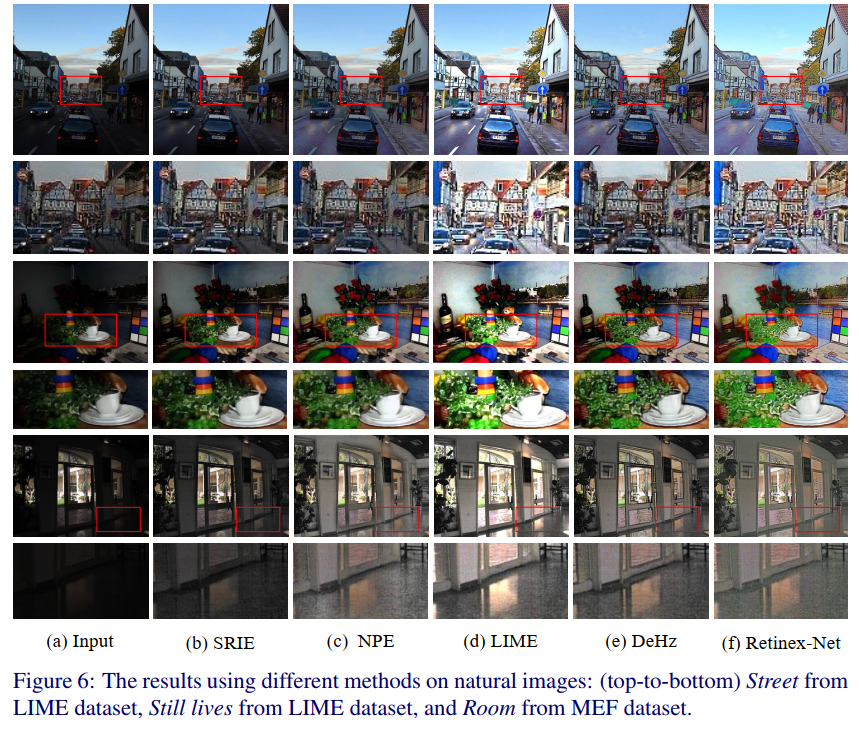## 4.4 微光增强和去噪联合